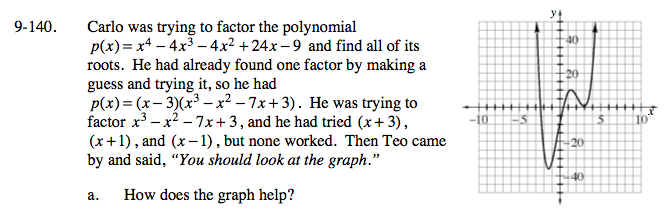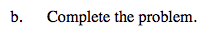### Home > A2C > Chapter Ch9 > Lesson 9.3.2 > Problem9-140

9-140.It shows us that 3 is a double root which means (x − 3)2 is a factor.Divide x3x2 − 7x + 3 by x − 3.

Use a multiplication rectangle to set it up.

Now use the quadratic formula to solve for x.

$x=3,-1\pm\sqrt{2}$